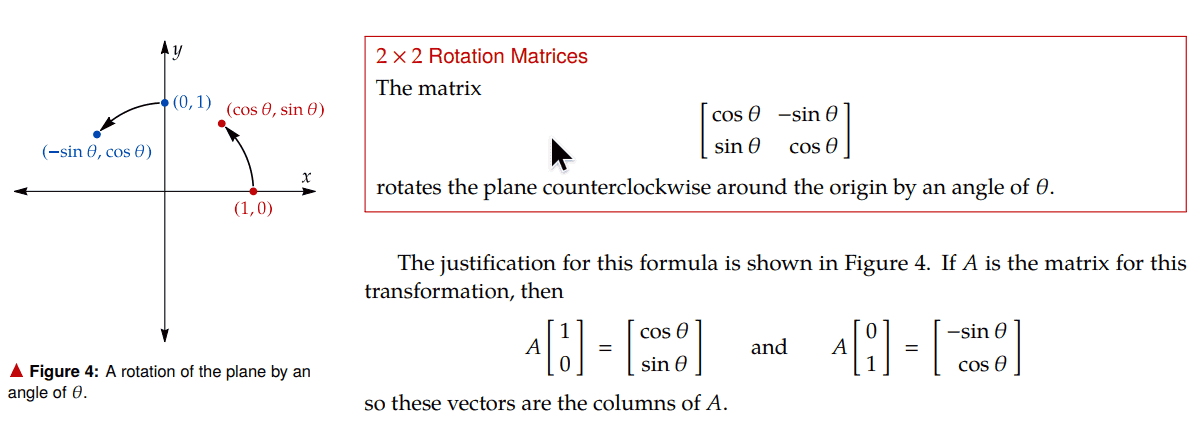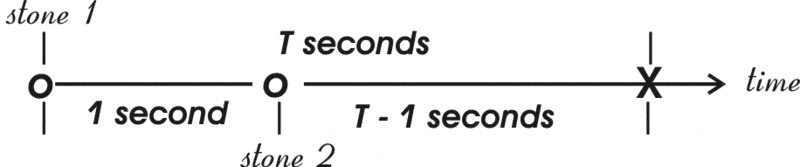# 072 is Q(theta) a linear transformation from R^2 to itself.

• MHB
Gold Member
MHB
if $Q(\theta)$ is

$\left[\begin{array}{rr} \cos{\theta}&- \sin{\theta}\\ \sin{\theta}&\cos{\theta} \end{array}\right]$

how is $Q(\theta)$ is a linear transformation from R^2 to itself.

ok I really didn't know a proper answer to this question but presume we would need to look at the unit circle

not sure if this helpsLast edited by a moderator:

Gold Member
MHB
Ok thanks
I usually don't get much replies on these linear algebra posts

skeeter
Ok thanks
I usually don't get much replies on these linear algebra posts

I'd help out, but it's been since my sophomore year in school (1973) since I've taken a course in Linear Algebra ... except for the very basic stuff, I haven't used it so I've "losed" it.

HOI
Better is to understand what "linear transformation" means! ANY transformation that can be written as a matrix multiplication is linear!

A transformation, L, on a vector space is "linear" if and only if
L(u+ v)= Lu+ Lv, for any vectors u and v, and
L(au)= aLu, for any vector u and scalar, a.

Here if $u= \begin{pmatrix}x \\ y \end{pmatrix}$ and $v= \begin{pmatrix} a \\ b\end{pmatrix}$, $L(u+ v)= \begin{pmatrix} cos(\theta) & -sin(\theta) \\ sin(\theta) & cos(\theta)\end{pmatrix}\begin{pmatrix}x+ a \\ y+ b\end{pmatrix}= \begin{pmatrix}(x+ a)cos(\theta)- (y+ b)sin(\theta) \\ (x+ a)sin(\theta)+ (y+ b)cos(\theta)\end{pmatrix}$.

While $Lu+ Lv= \begin{pmatrix} cos(\theta) & -sin(\theta) \\ sin(\theta) & cos(\theta)\end{pmatrix}\begin{pmatrix}x \\ y \end{pmatrix}+ \begin{pmatrix} cos(\theta) & -sin(\theta) \\ sin(\theta) & cos(\theta)\end{pmatrix}\begin{pmatrix} a \\ b \end{pmatrix}= \begin{pmatrix} xcos(\theta)- ysin(\theta) \\ xsin(\theta)+ y cos(\theta)\end{pmatrix}+ \begin{pmatrix} acos(\theta)- bsin(\theta) \\ asin(\theta)+ bcos(\theta)\end{pmatrix}= \begin{pmatrix}(x+ a)cos(\theta)- (y+ b)sin(\theta) \\ (x+ a)sin(\theta)+ (y+ b)cos(\theta)\end{pmatrix}$.

And \$L(au)= \begin{pmatrix} cos(\theta) & -sin(\theta) \\ sin(\theta) & cos(\theta)\end{pmatrix}\begin{pmatri
072 is Q(theta) is a linear transformation from R^2 to itself.
• New
• https://mathhelpboards.com/posts/124753/bookmark
https://mathhelpboards.com/data/avatars/s/0/55.jpg?1588030209
karush
Well-known member

Jan 31, 2012 2,838
if Q(θ)Q(θ) is

[cosθsinθ−sinθcosθ][cos⁡θ−sin⁡θsin⁡θcos⁡θ]

how is Q(θ)Q(θ) is a linear transformation from R^2 to itself.

ok I really didn't know a proper answer to this question but presume we would need to look at the unit circle

not sure if this helpsLast edited by a moderator: Today at 12:07 AM